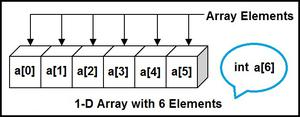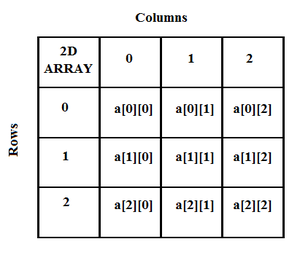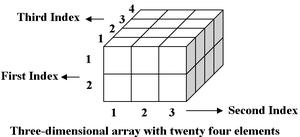# Calculation of address of element of 1-D, 2-D, and 3-D using row-major and column-major order

• Last Updated : 30 Sep, 2021

This article focuses on calculating the address of any element in a 1-Dimensional, 2-Dimensional, and 3-Dimensional array in Row major order and Column major order.

Calculating the address Of any element In the 1-D array: A 1-dimensional array (or single dimension array) is a type of linear array. Accessing its elements involves a single subscript that can either represent a row or column index.

Attention reader! Don’t stop learning now. Get hold of all the important DSA concepts with the DSA Self Paced Course at a student-friendly price and become industry ready.  To complete your preparation from learning a language to DS Algo and many more,  please refer Complete Interview Preparation Course.

In case you wish to attend live classes with experts, please refer DSA Live Classes for Working Professionals and Competitive Programming Live for Students.

Example:To find the address of an element in an array the following formula is used-

Address of A[I] = B + W * (I – LB)

I = Subset of element whose address to be found,
W = Storage size of one element store in any array(in byte),
LB = Lower Limit/Lower Bound of subscript(If not specified assume zero).

Example: Given the base address of an array A[1300…………1900] as 1020 and the size of each element is 2 bytes in the memory, find the address of A?

Solution:

Given:
Lower Limit/Lower Bound of subscript LB = 1300
Storage size of one element store in any array W = 2 Byte
Subset of element whose address to be found I = 1700

Formula:
Address of A[I] = B + W * (I – LB)

Solution:
Address of A = 1020 + 2 * (1700 – 1300)
= 1020 + 2 * (400)
= 1020 + 800

Calculate the address of any element in the 2-D array: The 2-dimensional array can be defined as an array of arrays. The 2-Dimensional arrays are organized as matrices which can be represented as the collection of rows and columns as array[M][N] where M is a number of rows and N is a number of columns.

Example:To find the address of any element in a 2-Dimensional array there are the following two ways-

• Row Major Order
• Column Major Order

Row Major Order: Row major ordering assigns successive elements, moving across the rows and then down the next row, to successive memory locations. In simple language, the elements of an array are being stored in a Row-Wise fashion.
To find the address of the element using row-major order use the following formula:

Address of A[I][J] = B + W * ((I – LR) * N + (J – LC))

I = Row Subset of an element whose address to be found,
J = Column Subset of an element whose address to be found,
W = Storage size of one element store in an array(in byte),
LR = Lower Limit of row/start row index of the matrix(If not given assume it as zero),
LC = Lower Limit of column/start column index of the matrix(If not given assume it as zero),
N = Number of column given in the matrix.

Example: Given an array, arr[1………10][1………15] with base value 100 and the size of each element is 1 Byte in memory. Find the address of arr with the help of row-major order?

Solution:

Given:
Storage size of one element store in any array W = 1 Bytes
Row Subset of an element whose address to be found I = 8
Column Subset of an element whose address to be found J = 6
Lower Limit of row/start row index of matrix LR = 1
Lower Limit of column/start column index of matrix = 1
Number of column given in the matrix N = Upper Bound – Lower Bound + 1
= 15 – 1 + 1
= 15

Formula:
Address of A[I][J] = B + W * ((I – LR) * N + (J – LC))

Solution:
Address of A = 100 + 1 * ((8 – 1) * 15 + (6 – 1))
= 100 + 1 * ((7) * 15 + (5))
= 100 + 1 * (110)

Column Major Order: If elements of an array are stored in column-major fashion means moving across the column and then to the next column then it’s in column-major order. To find the address of the element using column-major order uses the following formula:

Address of A[I][J] = B + W * ((J – LC) * M + (I – LR))

I = Row Subset of an element whose address to be found,
J = Column Subset of an element whose address to be found,
W = Storage size of one element store in any array(in byte),
LR = Lower Limit of row/start row index of matrix(If not given assume it as zero),
LC = Lower Limit of column/start column index of matrix(If not given assume it as zero),
M = Number of rows given in the matrix.

Example: Given an array arr[1………10][1………15] with base value 100 and the size of each element is 1 Byte in memory find the address of arr with the help of column-major order.

Solution:

Given:
Storage size of one element store in any array W = 1 Bytes
Row Subset of an element whose address to be found I = 8
Column Subset of an element whose address to be found J = 6
Lower Limit of row/start row index of matrix LR = 1
Lower Limit of column/start column index of matrix = 1
Number of column given in the matrix M = Upper Bound – Lower Bound + 1
= 10 – 1 + 1
= 10

Formula:
Address of A[I][J] = B + W * ((J – LC) * M + (I – LR))
Address of A = 100 + 1 * ((6 – 1) * 10 + (8 – 1))
= 100 + 1 * ((5) * 10 + (7))
= 100 + 1 * (57)

From the above examples, it can be observed that for the same position two different address locations are obtained that’s because in row-major order movement is done across the rows and then down to the next row, and in column-major order, first move down to the first column and then next column. So both the answers are right.

So it’s all based on the position of the element whose address to be found for some cases the same answers is also obtained with row-major order and column-major order and for some cases, different answers are obtained.

Calculate the address of any element in the 3-D Array: A 3-Dimensional array is a collection of 2-Dimensional arrays. It is specified by using three subscripts:

• Block size
• Row size
• Column size

More dimensions in an array mean more data can be stored in that array.

Example:To find the address of any element in 3-Dimensional arrays there are following two ways-

• Row Major Order
• Column Major Order

Row Major Order: To find the address of the element using row-major order, use the following formula:

Address of[i][j][k] = B + W * {[(I – LR) * N] +

[(J – LC)]} * R + [K – LB]

I = Row Subset of an element whose address to be found,
J = Column Subset of an element whose address to be found,
K = Block Subset of an element whose address to be found,
W = Storage size of one element store in any array(in byte),
LR = Lower Limit of row/start row index of matrix(If not given assume it as zero),
LC = Lower Limit of column/start column index of matrix(If not given assume it as zero),
LB = Lower Limit of blocks in matrix,
N = Number of column given in the matrix
R = Number of blocks given in the matrix.

Example: Given an array arr[1:9, -4:1, 5:10] with base value 400 and size of each element is 2 Bytes in memory find the address of element arr[-1] with the help of row-major order?

Solution:

Given:
Row Subset of an element whose address to be found I = 5
Column Subset of an element whose address to be found J = -1
Block Subset of an element whose address to be found K = 8
Storage size of one element store in any array(in Byte) = 2
Lower Limit of row/start row index of matrix LR = 1
Lower Limit of column/start column index of matrix LC = -4
Lower Limit of blocks in matrix LB = 5
Number of column given in the matrix N = Upper Bound – Lower Bound + 1
= 1 – (-4) + 1
= 6
Number of blocks given in the matrix R = Upper Bound – Lower Bound + 1
= 10 – 5 + 1
= 6

Formula:
Address of[i][j][k] = B + W * {[(I – LR) * N] + [(J – LC)]} * R + [K – LB]

Solution:
Address of[][][] = 400 + 2 * {[(5 – 1) * 6] + [(-1 + 4)]} * 6 + [8 – 5]
= 400 + 2 * ((4 * 6 + 3) * 6 + 3)
= 400 + 2 * (165)
= 730

Column Major Order: To find the address of the element using column-major order, use the following formula-

Address of[i][j][k] = B + W * {[(I – LR)] + [(J – LC) * M]} * R + [K – LB]

I = Row Subset of an element whose address to be found,
J = Column Subset of an element whose address to be found,
K = Block Subset of an element whose address to be found
W = Storage size of one element store in any array(in byte),
LR = Lower Limit of row/start row index of matrix(If not given assume it as zero),
LC = Lower Limit of column/start column index of matrix(If not given assume it as zero),
LB = Lower Limit of blocks in matrix,
M = Number of rows given in the matrix,
R = Number of blocks given in the matrix.

Example: Given an array arr[1:8, -5:5, -10:5] with base value 400 and size of each element is 4 Bytes in memory find the address of element arr with the help of column-major order?

Solution:

Given:
Row Subset of an element whose address to be found I = 3
Column Subset of an element whose address to be found J = 3
Block Subset of an element whose address to be found K = 3
Storage size of one element store in any array(in Byte) = 4
Lower Limit of row/start row index of matrix LR = 1
Lower Limit of column/start column index of matrix LC = -5
Lower Limit of blocks in matrix LB = -10
Number of rows given in the matrix M = Upper Bound – Lower Bound + 1
= 8 – 1 + 1
= 8
Number of blocks given in the matrix R = Upper Bound – Lower Bound + 1
= 5 + 10 + 1
= 16

Formula:
Address of[i][j][k] = B + W * {[(I – LR)] + [(J – LC) * M]} * R + [K – LB]

Solution:
Address of = 400 + 4 * {[(3 – 1)] + [3 + 5] * 8]} * 16 + [3 + 10]
= 400 + 4 * ((2 + 64) * 16 + 13)
= 400 + 4 * (1069)
= 400 + 4276
= 4676

My Personal Notes arrow_drop_up